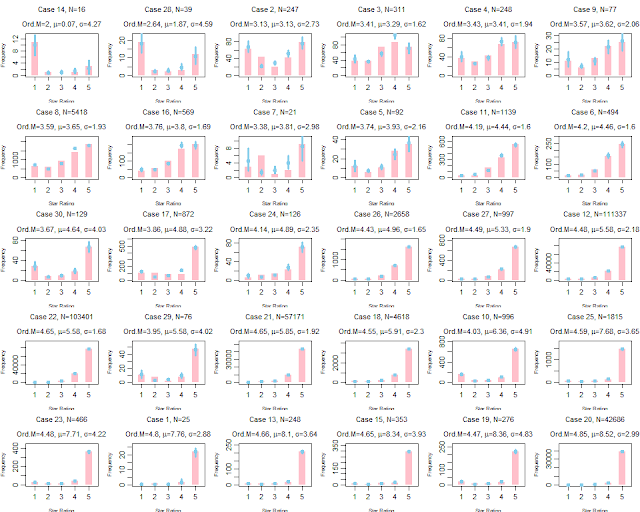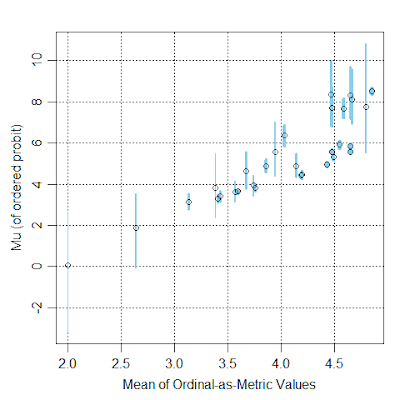## Monday, December 25, 2017

### Which movie is rated better? (Don't treat ordinal ratings as metric)

When deciding what movie to watch online, have you ever considered the star ratings provided by previous viewers? For example, Amazon.com has a 5-star rating system, in which reviewers can give a movie an ordinal rating from 1 star to 5 stars. Here are frequency histograms of 30 movies listed under "drama":Frequency histograms of star ratings from 30 movies (shown as pink bars). Posterior predictions of an ordered-probit model are shown by blue dots with blue vertical segments indicating the 95% HDIs.

Usually people analyze rating data as if the data were metric, that is, people pretend that 1 star is 1.0 on a metric scale, and 2 stars is 2.0 on the metric scale, and 3 stars is 3.0, and so forth. But this is not appropriate because all we know about the star ratings is their order, not their interval separation. The ordinal data should instead be described with an ordinal model. For more background, see Chapter 23 of DBDA2E, and this manuscript.

Here I used an ordered-probit model to describe the data from the 30 movies. I assumed the same response thresholds across the movies because the response scale is presented to everyone the same way, for all movies; this is a typical assumption. Each movie was given its own latent mean (mu) and standard deviation (sigma). I put no hierarchical structure on the means, as I didn't want the means of small-N movies to be badly shrunken toward enormous-N movies. But I did put hierarchical structure on the standard deviations, because I wanted some constraint on the sigma's of movies that show extreme ceiling effects in their data; it turns out the sigma's were estimated to vary quite a lot anyway.

Below is a graph of the resulting latent means (mu's) of the movies plotted against the means of their ordinal ratings treated as metric:Each point is a movie. Vertical axis is posterior mean (mu) of ordered probit model, with 95% HDI displayed as blue segment. Horizontal axis is mean of the star ratings treated as metric values.
In the scatter plot above, notice the many non-monotonicities; that is, as the means of ordinal-as-metric values increase along the horizontal axis, the latent mu's do not consistently increase on the vertical axis. In other words, the latent mu's are telling a different story than the ordinal-as-metric means.

Two movies with nearly equal ordinal-as-metric means, but with very different latent means in the ordered-probit model:Upper row shows ordered-probit fit; lower row shows t test with unequal variances. Notice the blue dots from the ordered-probit model fit the data much better than the blue normal distributions of the ordinal-as-metric model. (Case 19 is Ekaterina: The rise of Catherine the Great, and Case 26 is John Grisham's The Rainmaker.)
Do we conclude that the movies (above) are rated about the same, or that movie 19 is rated much better than movie 26? I think we have to conclude that movie 19 is rated much better than movie 26 because the ordered-probit model is a much better description of the data.

Two movies with ordinal-as-metric means that are significantly different in one direction but the latent means in the ordered-probit model are quite different in the opposite direction:Upper row shows ordered-probit fit; lower row shows t test with unequal variances. Notice the blue dots from the ordered-probit model fit the data much better than the blue normal distributions of the ordinal-as-metric model. (Case 10 is Miss Sloane, and Case 26 is John Grisham's The Rainmaker.)
Do we conclude that movie 26 is rated better than movie 10, or the other way around? I think we have to conclude that movie 10 is rated better than movie 26 because the ordered probit model is a much better description of the data.

This isn't (only) about movies: The point is that ordinal data from any source should not be treated as metric. Pretending that a rating of "1" is numeric 1.0, and rating "2" is 2.0, and rating "3" is 3.0, and so forth, is usually nonsensical because it's assuming metric information in the data that simply is not there. Treating the data as normally-distributed metric values is often a terrible description of the data. Instead, use an ordinal model for ordinal data. The ordinal model will describe the data better, and sometimes yield rather different implications than the ordinal-as-metric description.

For more information, see Chapter 23 of DBDA2E, and this manuscript titled, "Analyzing ordinal data with metric models: What could possibly go wrong?"

1.The ordered probit model assumes that there people's ratings come from a single underlying normal distribution. And on the wild West of the internet, one wonders if that is really true.

How well does it behave if, for example, people only vote the extreme values?

2.As in ANY modeling, there is no guarantee that a useful model is a correct model. But here the ordered probit is clearly a much more accurate descriptive model.

The ordered probit can produce all sorts of curious histograms, including extreme responses. The examples here are U shaped. See DBDA2E for trimodal, etc.

3.This is a nice application but still assumes the raters are calibrated. To avoid that assumption, you need to get within-person ratings, which incurs lots of missing values. The problem can then be treated as a large Stratified Cox Proportion Hazards model (e.g. partial rankings.)

4.Thanks, I've found these references quite helpful. However, after reading the manuscript linked above, I'm left wondering how to "properly" create scale scores from a set of ordinal items. Furthermore, if responses to the items were observed at the individual level, how might the scale scores be aggregated to the group level for subsequent analyses? Do you have any references to suggest? (I'm in the midst of analyzing the last bit of data for my dissertation and these issues are manifest :)

1."I'm left wondering how to "properly" create scale scores from a set of ordinal items." To clarify, in a Bayesian framework, preferably using R.

2.An example of a hierarchical model for ordinal data appears in this paper (linked below). Be sure to check its Appendix for the model specification.

https://papers.ssrn.com/sol3/papers.cfm?abstract_id=2519218